# Design of Reinforced Concrete Beams per ACI 318-05

Reinforced concrete is made of two materials, concrete and reinforcing steel.Concrete is made of five parts:
• Air
• Water
• Cement, five main types per ASTM
• Sand, fine aggregate
• Gravel, course aggregate
The compressive strength (fc’) of concrete is the 28-day strength. This could be from 2,500 psi to 20,000 psi. Most concrete used is between 3,000 psi to 6,000 psi. Concrete is very good in compression but its tensile strength is only about 8 to 15% of the compressive strength. This is the reason why we need reinforcing steel.When we load a beam, the bottom is in tension. Reinforcement could be fiber-reinforcement or reinforcing steel. In this course, we will only look at reinforcing steel. Reinforcing steel comes in the following sizes, areas, weights and diameters: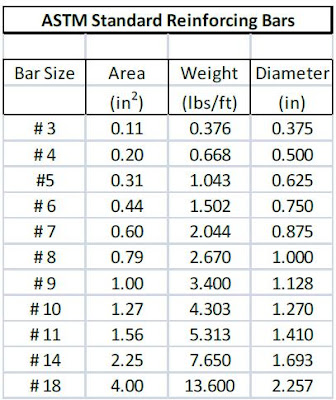Reinforcement steel comes in the followingdesignations,types, grades, strengths and available sizes.It appears from the above table, A615, grade 60 and A706, grade 60 cover all sizes.The stress distribution may be rectangular, parabolic, trapezoidal, etc. Here are two stress distributions, parabolic (b) and rectangular (c):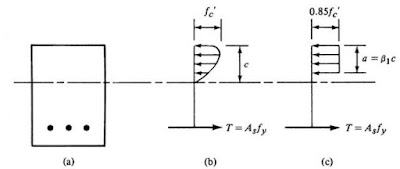We use figure c, rectangular. The ACI code says for concretes with fc’>4,000 psi, β can be determined with the following formula:
This is a table for the above formula (ACI 10.2.7.3):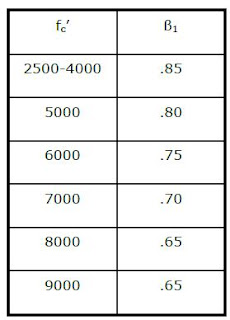The ACI code says design value must be greater than or equal to the required value.In the formula, ρ is the steel ratio, As / bd.Beams are considered to be under three types of control:
• Compression control, εt <0.002
• Transition, 0.002<εt<0.005
• Tension control, εt>0.005
We use a strength reduction factor to account for many uncertainties in the design.For tension-controlled beams, we use a strength reduction factor (φ) of 0.90.
ACI (10.5.1) specifies the minimum about of reinforcement by the following two formulas:
Note: When the code specifies a minimum and they give two or more formulas, we use the formula that yields the maximum. For example, if one formula gives 16 sq inch and the other formula gives 18 sq inch, then the minimum would be 18 sq inch. Also, bw is the width of the beam.
ACI (10.2.3) states that the maximum usable strain at extreme concrete compression fiber shall be assumed equal to 0.003. In other words, εc=0.003.It is desirable, under ordinary conditions, to design beams with a steel ration (ρ) between ρ min and ρ max.
Load factors are numbers, used to increase the estimated loads applied on a structure. The loads are increased to account for the uncertainties involved in estimating the magnitude of the loads. How good can you estimate the loads on the floor where you are right now? Sections 9.2 gives the required strength based on load factors and combinations of loads:Section 9 gives the following table for minimum depth of beams:In Section 7.7.1 of the code, it specifies the amount of cover for the reinforcement. Cover is the distance from the edge of the reinforcing bar to the face of the concrete beam. For beams with primary reinforcement, ties, stirrups and spirals, it is 1 ½ inches when the concrete is not exposed to weather or in contact with the ground.
In section 7.6 of the code, it specifies the minimum clear spacing between parallel bars in a layer to be db or 1”, whichever is larger. Remember, when the code specifies the minimum and gives you two or more items, you use the larger of the values. As a rule of thumb, beams 20-25 feet long have a ratio of d to b of 1.5 to 2. For longer beams the ratio of depth to width may be as high as 3-4. Beam dimensions are selected in whole inches. The width is usually a multiple of 2 or 3. Beams should probably not be less than 12” wide to get the steel and your hands in the form. For the usual situation, use bars of size # 11 and smaller if possible. Rarely will you use # 14 or # 18 bars.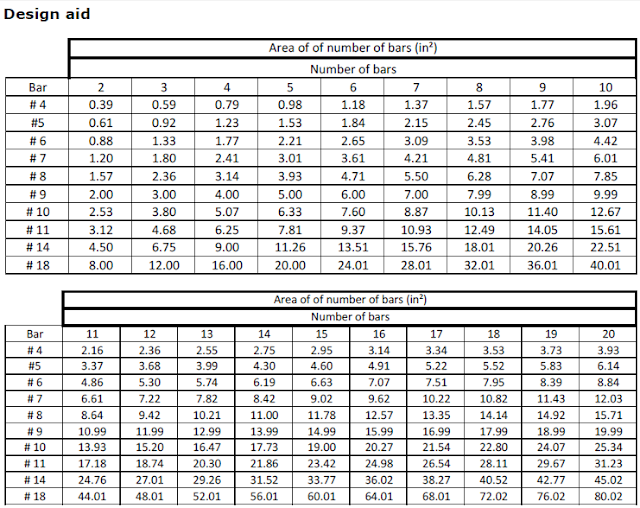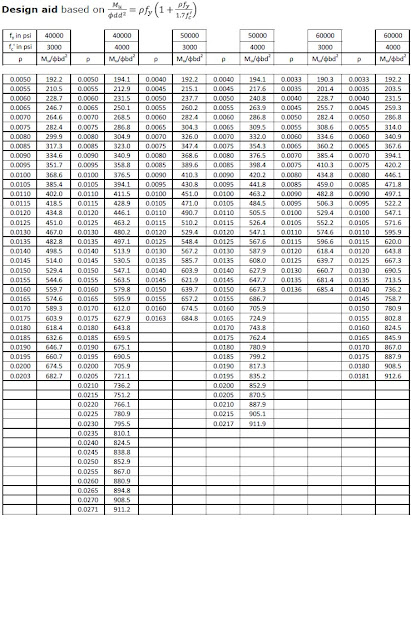[blogger]

Engineeersdaily

Name

Email *

Message *